# Plots of Data Collected over Time

Plot ordered data values collected over time in one of two ways that correspond to how the values are labeled.

• Run Chart: Label the data values from 1 to the number of values ordered by their time of collection.
• Time Series Chart: Label the data values by the time and/or date each data value was collected.

## Run Chart

Meaningful for sequentially ordered numerical data values such as by time, plot a run chart of a single variable according to the parameter run. Analogous to a time series visualization, the run chart plots the data values sequentially, but without dates or times. An analysis of the runs is also provided.

Illustrate with the lessR Employee data.

d <- Read("Employee")
##
## >>> Suggestions
##
## Data Types
## ------------------------------------------------------------
## character: Non-numeric data values
## integer: Numeric data values, integers only
## double: Numeric data values with decimal digits
## ------------------------------------------------------------
##
##     Variable                  Missing  Unique
##         Name     Type  Values  Values  Values   First and last values
## ------------------------------------------------------------------------------------------
##  1     Years   integer     36       1      16   7  NA  7 ... 1  2  10
##  2    Gender character     37       0       2   M  M  W ... W  W  M
##  3      Dept character     36       1       5   ADMN  SALE  FINC ... MKTG  SALE  FINC
##  4    Salary    double     37       0      37   53788.26  94494.58 ... 56508.32  57562.36
##  5    JobSat character     35       2       3   med  low  high ... high  low  high
##  6      Plan   integer     37       0       3   1  1  2 ... 2  2  1
##  7       Pre   integer     37       0      27   82  62  90 ... 83  59  80
##  8      Post   integer     37       0      22   92  74  86 ... 90  71  87
## ------------------------------------------------------------------------------------------

The data values for the variable Salary are not actually collected over time, but for illustration, here create a run chart of Salary as if the data were collected over time. The indices, the sequence of integers from 1 to the last data value, are created by Plot(). Only the data values are specified. Invoke the run parameter to instruct Plot() to plot the data in sequential order as a run chart.

Plot(Salary, run=TRUE)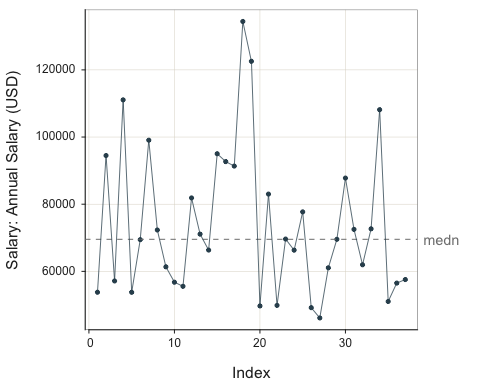## >>> Suggestions
## Plot(Salary, run=TRUE, size=0)  # just line segments, no points
## Plot(Salary, run=TRUE, lwd=0)  # just points, no line segments
## Plot(Salary, run=TRUE, fill="on")  # default color fill
##
##      n   miss         mean           sd          min          mdn          max
##      37      0    73795.557    21799.533    46124.970    69547.600   134419.230
##
## ------------
## Run Analysis
## ------------
##
## Total number of runs: 21
## Total number of values that do not equal the median: 36

The default run chart displays the plotted points in a small size with connecting line segments. Change the size of the points with the parameter size, here set to zero to remove the points entirely. Fill the area under the line segments with the parameter area_fill, here set to the default on but can express any color. Remove the center line with the parameter center_line set to off.

Plot(Salary, run=TRUE, size=0, area_fill="on", center_line="off")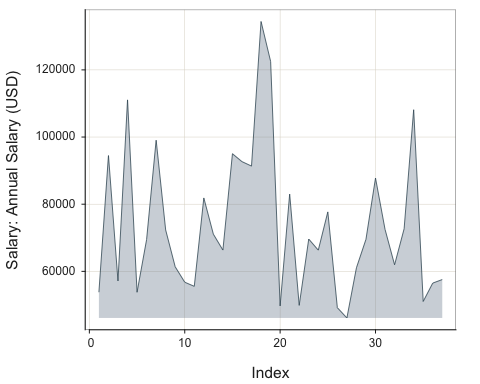## >>> Suggestions
## Plot(Salary, size=0, run=TRUE, area_fill="on", center_line="off", lwd=0, fill="on")  # just area
##
##      n   miss         mean           sd          min          mdn          max
##      37      0    73795.557    21799.533    46124.970    69547.600   134419.230
##
## ------------
## Run Analysis
## ------------
##
## Total number of runs: 21
## Total number of values that do not equal the median: 36

## Time Series Chart

Plot() can plot a time series from three different forms of the data:

• long-form
• wide-form
• time-series object

Plot() can also similarly plot a run chart in which it generates the index values sequentially ordered. A time series requires two variables, the time/date and each corresponding measured value to be plotted.

Plotting a variable of type Date as the x-variable in a scatterplot automatically creates a time series visualization. Plot() draws the connecting line segments, without the points at each time period (size=0). To add the area fill, for lessR set the area parameter to TRUE for the default color from the current color theme. Or, set to a specific color.

### Long-Format Data

Read time series data of stock Price for three companies: Apple, IBM, and Intel. The data table is in long form, part of lessR.

d <- Read("StockPrice")
##
## >>> Suggestions
##
## Data Types
## ------------------------------------------------------------
## character: Non-numeric data values
## Date: Date with year, month and day
## double: Numeric data values with decimal digits
## ------------------------------------------------------------
##
##     Variable                  Missing  Unique
##         Name     Type  Values  Values  Values   First and last values
## ------------------------------------------------------------------------------------------
##  1      date      Date   1350       0     450   1985-01-01 ... 2022-06-01
##  2   Company character   1350       0       3   Apple  Apple ... Intel  Intel
##  3     Price    double   1350       0    1331   0.10105  0.086241 ... 44.071625  43.389999
## ------------------------------------------------------------------------------------------
d[1:5,]
##         date Company    Price
## 1 1985-01-01   Apple 0.101050
## 2 1985-02-01   Apple 0.086241
## 3 1985-03-01   Apple 0.077094
## 4 1985-04-01   Apple 0.074045
## 5 1985-05-01   Apple 0.060543

Activate a time series plot by setting the $$x$$-variable to a variable of R type Date, which is true of the variable date in this data set. Can also plot a time series by passing a time series object, created with the base R function ts() as the variable to plot.

Here plot just for Apple, with the two variables date and Price, stock price. The parameter rows specifies what rows of the input data frame to retain for the analysis.

Plot(date, Price, rows=(Company=="Apple"))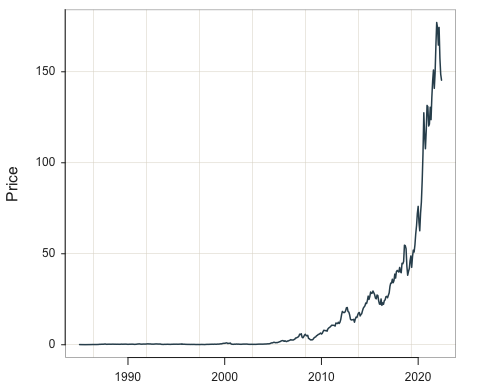## --- Pearson's product-moment correlation ---
##
## Number of paired values with neither missing, n = 450
## Sample Correlation of date and Price: r = 0.655
##
## Hypothesis Test of 0 Correlation:  t = 18.347,  df = 448,  p-value = 0.000
## 95% Confidence Interval for Correlation:  0.599 to 0.705
## 

Here, add the default fill color by setting the area_fill parameter to "on". Can also specify a custom color.

Plot(date, Price, rows=(Company=="Apple"), area_fill="on")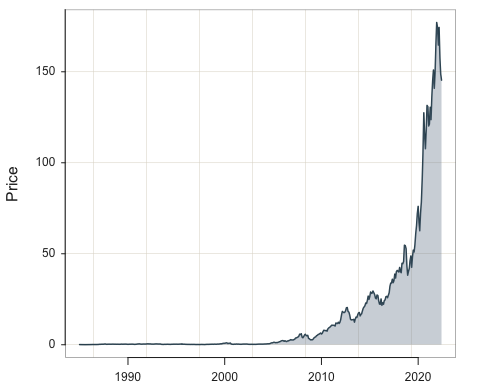## --- Pearson's product-moment correlation ---
##
## Number of paired values with neither missing, n = 450
## Sample Correlation of date and Price: r = 0.655
##
## Hypothesis Test of 0 Correlation:  t = 18.347,  df = 448,  p-value = 0.000
## 95% Confidence Interval for Correlation:  0.599 to 0.705
## 

With the by parameter, plot all three companies on the same panel.

Plot(date, Price, by=Company)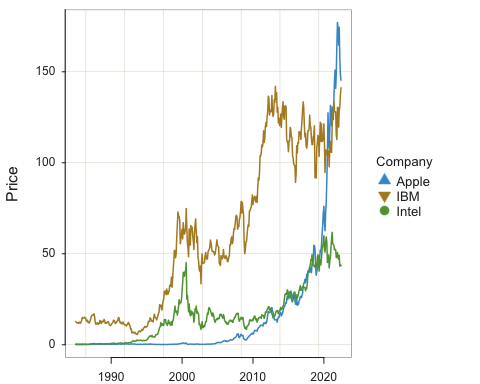Stack the plots by setting the parameter stack to TRUE.

Plot(date, Price, by=Company, stack=TRUE)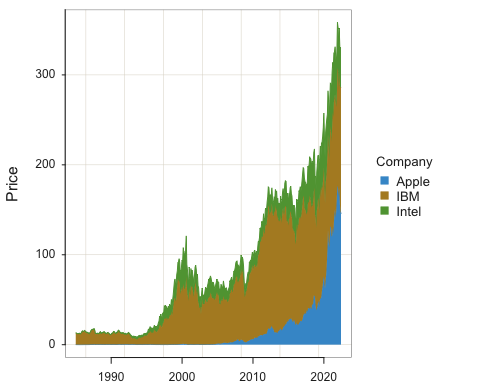With the by1 parameter, plot all three companies on the different panels, a Trellis plot.

Plot(date, Price, by1=Company)
## [Trellis graphics from Deepayan Sarkar's lattice package]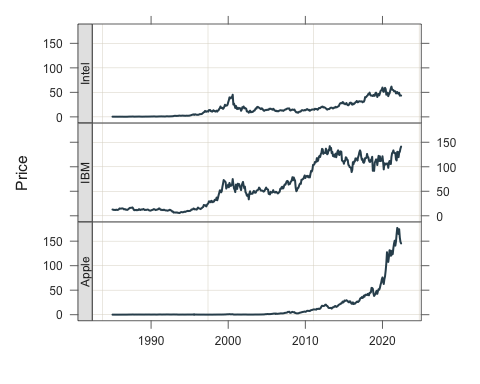Do the Trellis plot with some color. Learn more about customizing visualizations in the vignette utlities.

style(sub_theme="black", window_fill="gray10")
Plot(date, Price, by1=Company, n_col=1, fill="darkred", color="red", trans=.55)
## [Trellis graphics from Deepayan Sarkar's lattice package]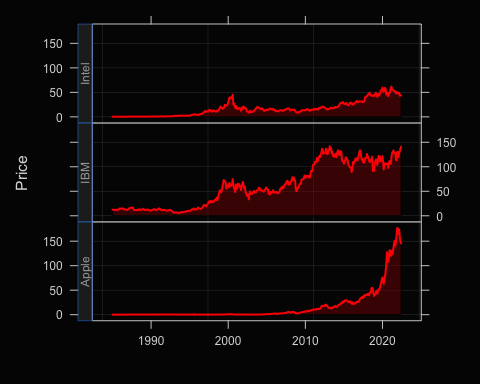Return to the default style, then turn off text output for subsequent analyses.

style()
## theme set to "colors"
style(quiet=TRUE)

Set a baseline of 25 with the area_origin parameter for a Trellis plot, with default fill color.

Plot(date, Price, by1=Company, xlab="", area_fill="on", area_origin=25)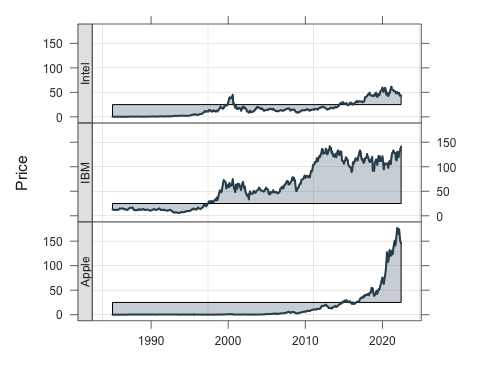Change the aspect ratio with the aspect parameter defined as height divided by width.

Plot(date, Price, by1=Company, aspect=.5, area_fill="slategray3")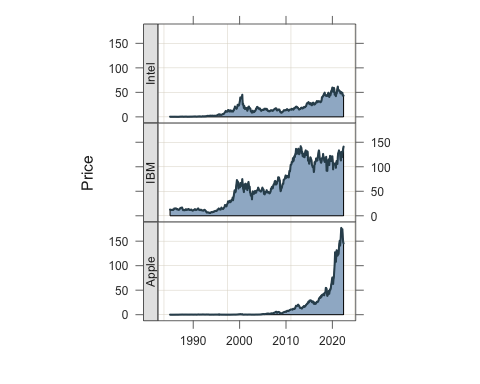Stack the three time series, fill under each curve with a version of the lessR sequential range "emeralds".

Plot(date, Price, by=Company, trans=0.4, stack=TRUE, area_fill="emeralds")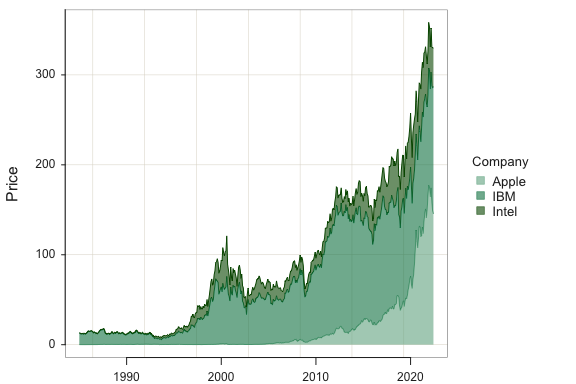### Wide-Format Data

Plot() also reads wide-format data. We have no available wide form time data with lessR, so first convert the long form as read to the wide form. In the wide form, the three companies each have their own column of data, repeated for each date. Use the lessR function reshape_wide() to do the conversion.

dw <- reshape_wide(d, group="Company", response="Price", ID="date")
head(dw)
##         date    Apple      IBM    Intel
## 1 1985-01-01 0.101050 12.71885 0.379217
## 2 1985-02-01 0.086241 12.49734 0.345303
## 3 1985-03-01 0.077094 11.94072 0.342220
## 4 1985-04-01 0.074045 11.89371 0.339137
## 5 1985-05-01 0.060543 12.09351 0.325263
## 6 1985-06-01 0.062720 11.73814 0.320639

Now the analysis, which repeats a previous analysis, but with wide-form data. Because the data frame is not the default d, explicitly indicate with the data parameter.

Plot(date, c(Intel, Apple, IBM), area_fill="blues", stack=TRUE, trans=.4, data=dw)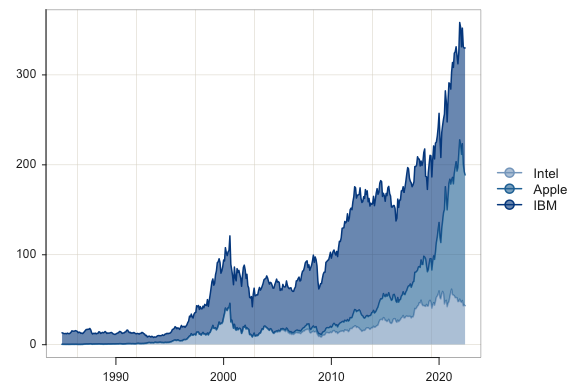### Time-Series Object Data

Can also plot directly from an R time series object, created with the base R ts() function.

a1.ts <- ts(dw\$Apple, frequency=12, start=c(1980, 12))
Plot(a1.ts)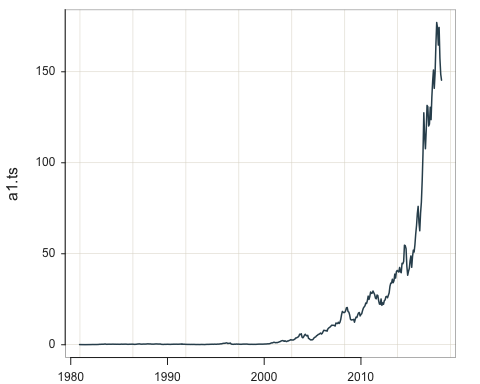With style() many themes can be selected, such as "lightbronze", "dodgerblue", "darkred", and "gray" for gray scale. When no theme or any other parameter value is specified, return to the default theme, colors.

style()

## Annotation

The annotations in the following visualization consist of the text field “iPhone” with an arrowhead that points to the time that the first iPhone became available. With lessR, list each component of the annotation as a vector for add. Any value listed that is not a keyword such as “rect” or “arrow” is interpreted as a text field. Then, in order of their occurrence in the vector for add, list the needed coordinates for the objects. To place the text field “iPhone” requires one coordinate, <x1,y1>. To place an “arrow” requires two coordinates, <x1,y1> and <x2,y2>. For example, the second element of the y1 vector is the y1 value for the “arrow”. The text field does not require a second coordinate, so specify x2 and y2 as single elements instead of vectors.

x <- as.Date("2007-06-01")
Plot(date, Price, rows=(Company == "Apple"), fill="on",
x1=c(x,x), y1=c(100,90), x2=x, y2=30)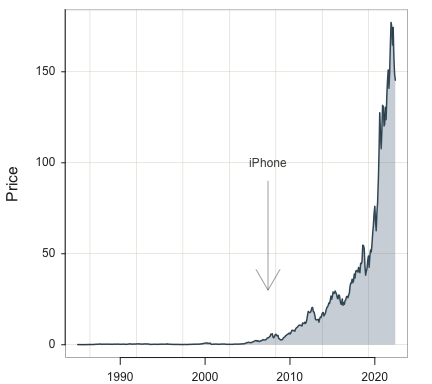## Full Manual

Use the base R help() function to view the full manual for Plot(). Simply enter a question mark followed by the name of the function.

?Plot

## More

More on Scatterplots, Time Series plots, and other visualizations from lessR and other packages such as ggplot2 at:

Gerbing, D., R Visualizations: Derive Meaning from Data, CRC Press, May, 2020, ISBN 978-1138599635.